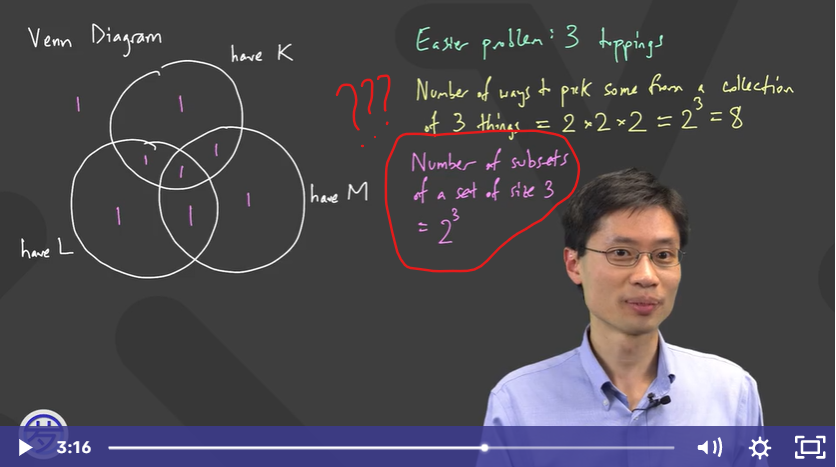# Why bother having to say it as "Number of subsets of a set of size 3" When we can just say "2 to the power of 3"? Which is easier?

•• Hi @victorioussheep ! It's true that "number of subsets of a set of size 3" might be a bit of a mouthful compared to just "2^3". That's one of the nice things about math-- it simplifies sometimes confusing words into easier expressions! But in the video's case, the "number of subsets of a set of size 3" comes from what we want to find: we want to know the number of topping choices where we have at least one of (ketchup, mustard, lettuce) on the burger. So the "set of size 3" is the set (ketchup, mustard, lettuce), and the "subset" is a particular topping choice from there. We are counting up how many subsets there are. And that amount equals 2^3, as Prof. Loh explained in the video.

So although the first expression is a bit long and maybe convoluted, it's helpful since it clarifies what exactly we're trying to solve, and 2^3 is the answer to that question. If the first wordy expression comes intuitively to you, though, at that point you can skip over it!

Hope this could clarify!